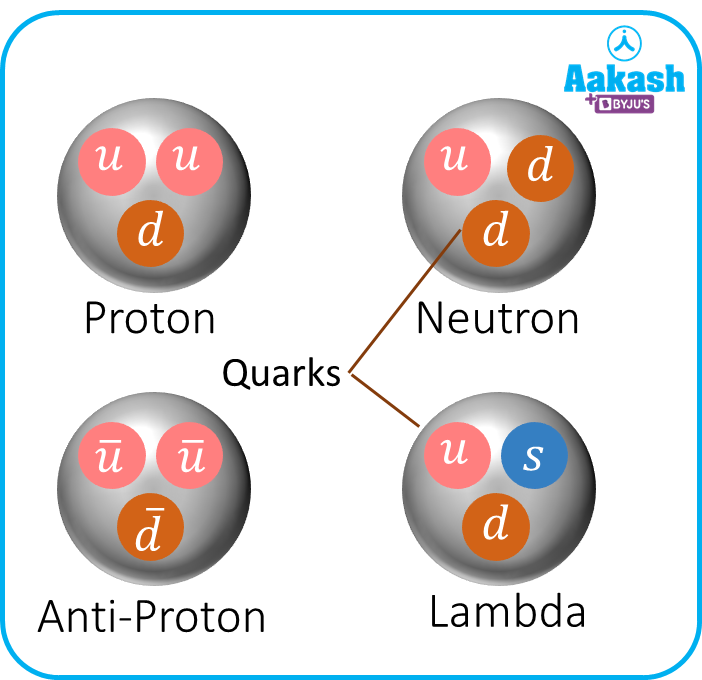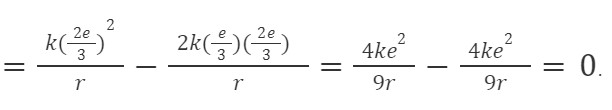• Call Now

1800-102-2727•

# Quark-Definition, Types, Practice problems, FAQs

You might have heard about the fundamental subatomic particles which make up matter– protons, electrons and neutrons. As more research went into studying subatomic particles, it was later discovered that thousands of them make up the universe. For instance, muons, which weigh about 207 times as much as that of an electron, are found in almost every corner of the universe. They are formed when cosmic rays collide with atoms in the Earth’s atmosphere. Subatomic particles have charge, mass, color and spin. Quarks were discovered by Murray Gell-Mann and George Zweig in 1964. They are elementary particles which upon combination form hadrons–a class of particles to which protons and electrons belong to. For every particle there is an antiparticle which has the same mass but different charge. For instance, the antiparticle of electron is positron with a charge of +e. In this article, we will explore quarks in detail.

• Definition of quark
• Types of quark
• Practice problems
• FAQs

## Definition of quark

A quark is said to be a fundamental constituent of matter. It is an elementary particle. Two quarks combine together to form a hadron. The most stable hadrons are protons and neutrons. It carries a spin of $\frac{1}{2}$ and its baryon number is $\frac{1}{3}.$ Quark is denoted by a symbol q. Examples: protons consist of two up quarks and one down quark. A neutron on the other hand, is made of two down quarks and one up quark. It is found that quarks cannot exist alone; according to the Standard theoretical Model.

## Types of quark

The three main types of quark are: Up quark , Down quark, strange quark. The corresponding antiparticle for a quark is called an Antiquark–they have the same mass, spin and even lifetime. The whole universe is made of 12 fundamental particles– six quarks and six leptons.The six types of quarks are: up, down, top, bottom, strange and charm.

Up quark

They are the lightest amongst all quarks, and also the most stable due to their lowest mass.

The symbol for quark is u and that of the anti-quark is u . The mass of the quark ranges from 1.7 to 3.1 MeV/c2. It carries a charge of 23e;e- electronic charge.

Down quark

The down quark is denoted by d, and the antiquark equivalent is denoted by d. Its mass lies between 4.1 to 5.7 MeV/c2. It carries a charge of $-\frac{1}{3}e.$Strange quark

The strange quark is denoted by s. Its antiparticle is denoted by s . It carries a charge of $-\frac{1}{3}e.$

The charm quark

The charm quark is denoted by c. Its antiparticle is denoted by c . It carries a charge of $+\frac{2}{3}e$

The top quark

The top quark carries a symbol t and its antiparticle is denoted by t. The mass of top quark is 172.9 + 1.5 GeV/c2, and its charge is $+\frac{2}{3}e$

The bottom quark

The bottom quark carries a symbol b and its antiquark is denoted by b . The mass of the bottom quark is about 4.1 GeV/c2. It carries a charge of $-\frac{1}{3}e.$

## Practice problems

Q.It is a known fact that protons and neutrons are made of elementary particles called quarks. Suggest a possible quark composition of a proton and a neutron.

A.

There are two types of quarks:

Up quark $\to \frac{2e}{3}$

Down quark $\to -\frac{1e}{3}$

Now proton,

2 up quarks and 1 down quark.

Neutron charge $=0=\left(\frac{2}{3}-\frac{1}{3}-\frac{1}{3}\right)e=0.$

2 down quarks and 1 up quark.

Q.Quarks are

1. Atomic particles
2. Nuclear particles
3. Subatomic particles
4. None of the above

A. c

Quarks are subatomic particles.

Q. In the quark model, a proton is composed of three quarks (2 up quarks, 1 down quark). An Up quark has a charge of +2e3 and a down quark has a charge of -e3. Three quarks are equidistant from each other at a distance of r. The total electrostatic potential energy of the system is

(a) $\frac{4K{e}^{2}}{9r}$ (b)$\frac{8K{e}^{2}}{9r}$ (c)Zero (d)$\frac{2K{e}^{2}}{3r}$

A. c

${q}_{1}=\left(\frac{2e}{3}\right);{q}_{2}=\left(\frac{2e}{3}\right);{q}_{3}=-\left(\frac{e}{3}\right)$

Electrostatic potential energy, $\mathrm{\Sigma }\frac{k{q}_{i}{q}_{j}}{{r}_{ij}}=\frac{k{q}_{1}{q}_{2}}{r}+\frac{k{q}_{2}{q}_{3}}{r}+\frac{k{q}_{1}{q}_{3}}{r}$Q. The atomic nucleus contains

(i) protons (ii) neutrons (iii) quarks (iv) leptons

(a)(i),(ii) (b)(i),(ii),(iii),(iv) (c) (i),(ii),(iv) (d)(i),(ii),(iii)

A. d

The atomic nucleus is made of neutrons, protons and quarks.

## FAQs

Q. How long does a quark last?
A.
A quark has a short life span and lasts about 510-25 s.

Q. Can quarks be broken down?
A.
No, quarks cannot be broken down, instead, they only undergo color change.

Q. How are quarks formed?
A.
After the event of the big bang, the universe cooled down and gave rise to quarks.

Q.Do quarks have mass?
A.
Yes, quarks have a wide range of masses. For instance, top quarks, t are about 180 times heavier than a proton.Talk to our expert
Resend OTP Timer =
By submitting up, I agree to receive all the Whatsapp communication on my registered number and Aakash terms and conditions and privacy policy